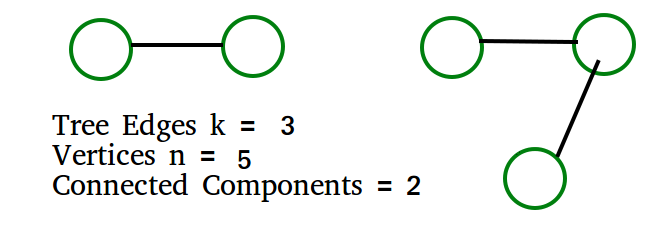# GATE | Gate IT 2005 | Question 14

In a depth-first traversal of a graph G with n vertices, k edges are marked as tree edges. The number of connected components in G is
(A) k
(B) k + 1
(C) n – k – 1
(D) n – k

Explanation: Tree edges are the edges that are part of DFS tree.  If there are x tree edges in a tree, then  x+1 vertices in the tree.

The output of DFS is a forest if the graph is disconnected.  Let us see below simple example where graph is disconnected.The above example matches with D option

More Examples:

1) All vertices  of Graph are connected.  k must be n-1.  We get number of connected components  = n- k =  n – (n-1) = 1

2) No vertex is connected. k must be 0.  We get number of connected components  = n- k =  n – 0 = n

Quiz of this Question

My Personal Notes arrow_drop_up
Article Tags :

Be the First to upvote.

Please write to us at contribute@geeksforgeeks.org to report any issue with the above content.